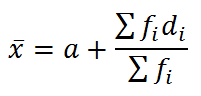# Assumed Mean Method

In statistics, the assumed mean method is used to calculate mean or arithmetic mean of a grouped data. If the given data is large, then this method is recommended rather than a direct method for calculating mean. This method helps in reducing the calculations and results in small numerical values. This method depends on estimating the mean and rounding to an easy value to calculate with. Again this value is subtracted from all the sample values. When the samples are converted into equal size ranges or class intervals, a central class is chosen and the computations are performed.

## Assumed Mean Method Formula

Let x1, x2, x3,…,xn are mid-points or class marks of n class intervals and f1, f2, f3, …, fn are the respective frequencies. The formula of the assumed mean method is:Here,

a = assumed mean

fi = frequency of ith class

di = xi – a = deviation of ith class

Σfi = n = Total number of observations

xi = class mark = (upper class limit + lower class limit)/2

Relation Between Mean Median and Mode

Statistics For Class 10

Statistics Class 11

## Assumed Mean Method Questions

If xi and fi are numerically large, the assumed mean method is preferred. Below are some examples of calculating the mean of grouped data by this method.

Example 1:

The following table gives information about the marks obtained by 110 students in an examination.

 Class 0-10 10-20 20-30 30-40 40-50 Frequency 12 28 32 25 13

Find the mean marks of the students using the assumed mean method.

Solution:

 Class (CI) Frequency (fi) Class mark (xi) di = xi – a fidi 0-10 12 5 5 – 25 = – 20 -240 10-20 28 15 15 – 25 = – 10 -280 20-30 32 25 = a 25-25 = 0 0 30-40 25 35 35-25 = 10 250 40-50 13 45 45-25 = 20 260 Total Σfi =110 Σfidi = -10

Assumed mean = a = 25

Mean of the data:= 25 + (-10/ 110)

= 25 -( 1/11)

= (275-1)/11

= 274/11

=24.9

Hence, the mean marks of the students are 24.9.

Example 2:

The table below gives information about the percentage distribution of female employees in a company of various branches and a number of departments.

 Percentage of female employees Number of departments 5-15 1 15-25 2 25-35 4 35-45 4 45-55 7 55-65 11 65-75 6

Find the mean percentage of female employees by the assumed mean method.

Solution:

 Percentage of female employees (CI) Number of departments (fi) Class mark (xi) di = xi – a fidi 5-15 1 10 -30 -30 15-25 2 20 -20 -40 25-35 4 30 -10 -40 35-45 4 40 = a 0 0 45-55 7 50 10 70 55-65 11 60 20 220 65-75 6 70 30 180 Total Σfi =35 Σfidi = 360

Assumed mean = a = 40

Mean = a+ (Σfidi /Σfi)

=40+ (360/35)

= 40+(72/7)

= 40 + 10.28

=50.28 (approx)

Hence, the mean percentage of female employees is 50.28.

1. Thank you sir/ma for this, I learnt alot Im grateful. I have a question Is the assumed mean always centrally placed in the class mark?. Thank you.

1. MPASWIN

no need of it but if u take the central value it would b easier 4 calculation

2. This website is really good you can even have a with an academic advisor if you are still confused

3. Thankyou,this helped me a lot!!

4. Medha Shankari

Good website. Nice. I understood clearly.

5. Student

Thank u so much
Thank u for easy and many examples too

6. Balogun david

Nice one thanks

7. Thank you, this helped a lot :)))

8. Thank you a lot. This is really helpful 🙂